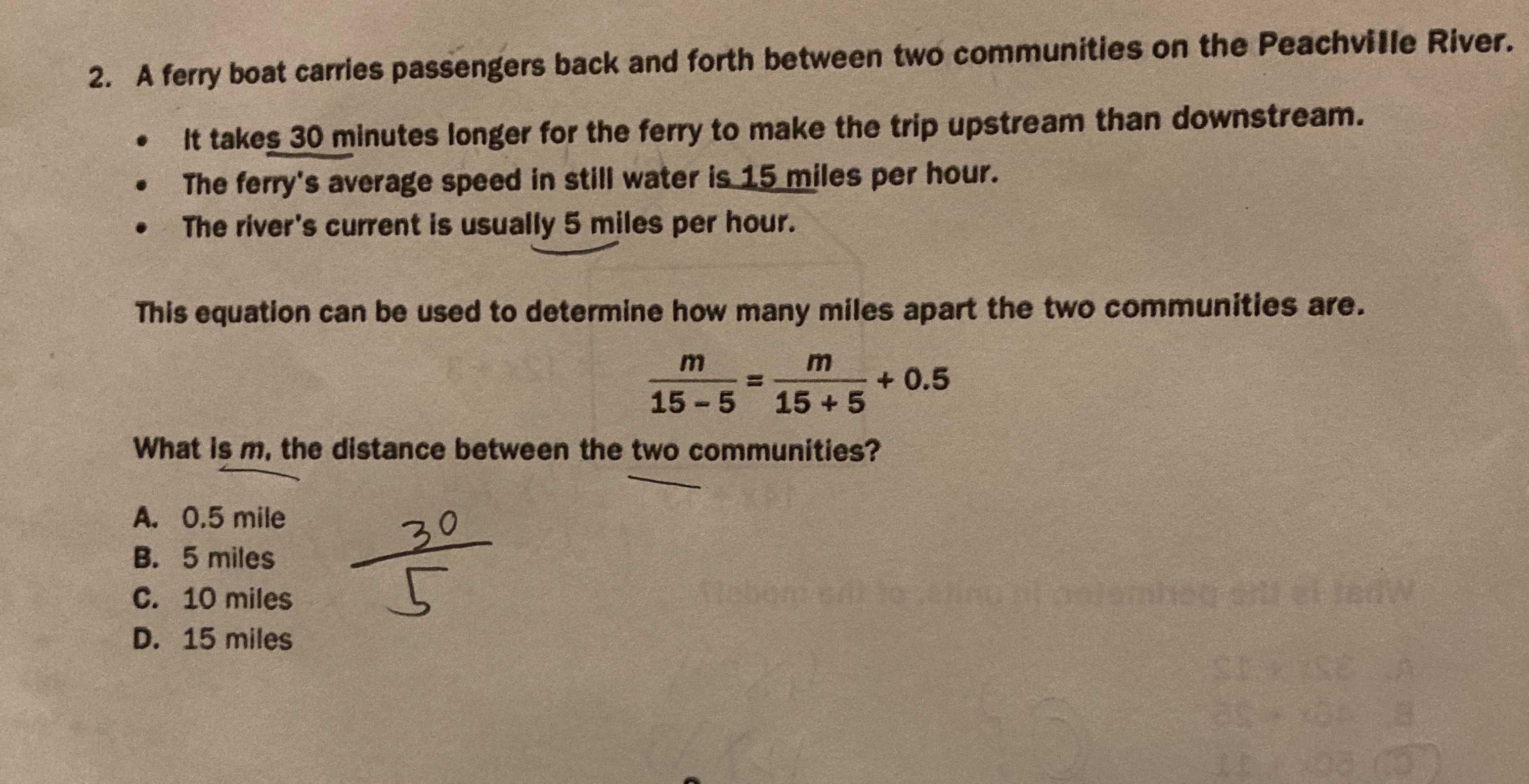### Still have math questions?

Algebra
Question2. A ferry boat carries passengers back and forth between two communities on the Peachville River. - It takes $$30$$ minutes longer for the ferry to make the trip upstream than downstream. - The ferry's average speed in still water is $$15$$ miles per hour. This equation can be used to determine how many miles apart the two communities are.

What is $$m$$ , the distance between the two communities? A. $$0.5$$ mile B. $$5$$ miles C. $$10$$ miles D. $$15$$ miles